# 9.2. N-BODY PROBLEM

Parallel Computing on Heterogeneous Networks, by Alexey Lastovetsky
ISBN 0-471-22982-2 Copyright 2003 by John Wiley & Sons, Inc.

## 9.1. LINEAR ALGEBRA

### 9.1.1. Matrix Multiplication

Matrix multiplication is widely used in a variety of applications and is often one of the core components of many scientific computations. It is a simple but very important linear algebra kernel. We have widely used matrix multiplication in this book. We used it to analyze the scalability of different parallel architectures, including shared memory multiprocessors (Section 3.1), distributed memory multiprocessors (Section 4.1), and computer networks (Section 5.2). We used matrix multiplication to illustrate parallel programming languages and tools such as MPI 1.1 (Section 4.2.6), HPF 1.1 (Section 4.3), HPF 2.0 (Section 5.1.1), and mpC (Sections 6.10 and 7.1).

In this section we start by introducing a more realistic parallel algorithm for matrix multiplication on homogeneous distributed memory multiprocessors than those given in Section 4.1. Then we give a modification of this algorithm for heterogeneous computer networks, its implementation in the mpC language, and some experimental results.

9.1.1.1. Block Cyclic Algorithm of Parallel Matrix Multiplication on Homogeneous Platforms. The way in which matrices are distributed over the processors has a major impact on the performance and scalability of the parallel algorithm. In Section 4.1 we considered two distributions, both known as block distributions. We demonstrated that they are equivalent in terms of computation cost. We also demonstrated that the communication cost of the parallel algorithm based on the two-dimensional block distribution is considerably less than that of the parallel algorithm based on the one-dimensional distribution. Therefore we take this two-dimensional algorithm as a basis for further improvements.

In our performance analysis we proceeded from the assumption that the parallel algorithm consists of two basic steps:

• First all processors communicate so that each processor receives all data necessary to compute its block of the resulting matrix (shown shaded in gray in Figure 4.3);

• Then, all processors compute the resulting matrix in parallel.

The scalability of this large-grained parallel algorithm in terms of per-processor computation cost is actually far from the ideal situation presented in Section 4.1. Recall that we assumed that the per-processor computation cost of the parallel multiplication of two dense square n x n matrices A and B on a p-processor MPP is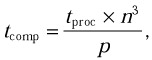where tproc is a constant representing the speed of a single processor and does not depend on n and p.

In reality, tproc is not constant. It depends on the size of data simultaneously stored in the memory of each individual processor and used by the processor in computations. The memory typically has a hierarchical structure with levels of fixed sizes (see Section 2.7 for more detail). Therefore, as more processed data are stored in the memory, the more levels of the memory hierarchy they fill. As a result more data become stored in slow memory. This increases the average execution time of a single arithmetic operation. Thus tproc is an increasing function of the size of stored (and processed) data.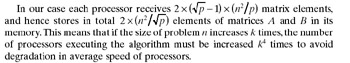To improve the scalability and per-processor computation cost of the basic two-dimensional block algorithm, we modify it as follows: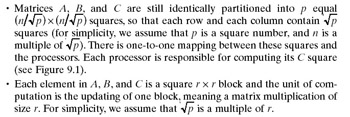• The algorithm consists of n/r steps. At each step k,

• a column of blocks (the pivot column) of matrix A is communicated (broadcast) horizontally (see Figure 9.1),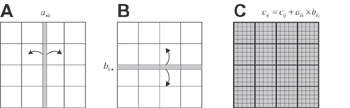Figure 9.1: One step of the modified algorithm of parallel matrix-matrix multiplication based on two-dimensional block distribution of matrices A, B, and C. First, the pivot column a•k of r x r blocks of matrix A (shown shaded in gray) is broadcast horizontally, and the pivot row bk• of r x r blocks of matrix B (shown shaded in gray) is broadcast vertically. Then each r x r block cij of matrix C (also shown shaded in gray) is updated, cij = cij + aik x bkj.

• a row of blocks (the pivot row) of matrix B is communicated (broadcast) vertically (see Figure 9.1), and

• each processor updates each block in its C square with one block from the pivot column and one block from the pivot row so that each block cij(i, j {1,..., n/r}) of matrix C will be updated, cij = cij + aik x bkj (see Figure 9.1).

Thus, after n/r steps of the algorithm, each block cij of matrix C will bethat is, C = A x B.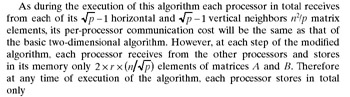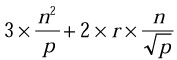elements of matrices A, B, and C in its memory, while at any execution time of the basic two-dimensional algorithm, each processor stores elements of these matrices. The significant decrease in the amount of data, which are simultaneously stored and processed by each individual processor, leads to a lower per-processor computation cost and better scalability of the algorithm. Now, if the size of the problem increases k times, then we will need to increase the number of processors executing the algorithm only k2 times instead of k4 times in order to avoid degradation in the average speed of processors. Optimal values of r depend on the memory hierarchy. Usually r is much smaller than p, r << p.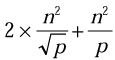Note that at each step k, each processor Pij participates in two collective communication operations: a broadcast involving the row of processors Pi• and a broadcast involving the column of processors P•j. Processor PiK is the root for the first broadcast, and processor PKj is the root for the second. As r is usually much less than m, in most cases at the next step k + 1 of the algorithm, processor PiK will be again the root of the broadcast involving the row of processors Pi•, and processor PKj will be the root of the broadcast involving the column of processors P•j. Therefore, at step k + 1, the broadcast involving the row of processors P•j cannot start until processor PiK completes this broadcast at step k. Similarly the broadcast involving the column of processors P•j cannot start until processor PKj completes that broadcast at step k. The root of the broadcast communication operation completes when its communication buffer can be re-used. Typically the completion means that the root has sent out the contents of the communication buffer to all receiving processors.

The strong dependence between the successive steps of the parallel algorithm hinders a parallel execution of the steps. If at the successive steps of the algorithm the broadcast operations involving the same set of processors had different roots, they could be executed in parallel. As a result more communications will be executed in parallel, and more computations and communications will be overlapped.

To break the dependence between the successive steps of the algorithm, we modify the way in which matrices A, B, and C are distributed over the processors. The modified distribution is called a two-dimensional block cyclic distribution. It can be summarized as follows:

• Each element in A, B, and C is a square r x r block.

• The blocks are scattered in a cyclic fashion along both dimensions of the m x m processor grid so that for all i, j {1,..., n/r}, blocks aij, bij, cij will be mapped to processor PIJ where I = (i - 1) mod m + 1 and J = (j - 1) mod m + 1.

Figure 9.2a illustrates the distribution from the matrix’s point of view. The matrix is now partitioned into n2/(r2 x m2) equal squares so that each row and each column contain n/(r x m) squares. All of the squares are identically partitioned into m2 equal r x r blocks, so that each row and each column contain m blocks. There is one-to-one mapping between these blocks and the processors. Thus all of the m x m squares are identically distributed over the m x m processor grid in a two-dimensional block fashion. Figure 9.2b shows this distribution from the processor’s point of view. Each square represents the total area of blocks allocated to a single processor.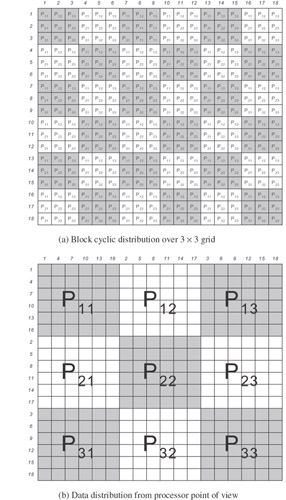Figure 9.2: A matrix with 18 x 18 blocks is distributed over a 3 x 3 processor grid. The numbers at the left and at the top of the matrix represent indexes of a row of blocks and a column of blocks, respectively. (a) The labeled squares represent blocks of elements, and the label indicates at which location in the processor grid the block is stored—all blocks labeled with the same name are stored in the same processor. Each shaded and unshaded area represents different generalized blocks. (b) Each processor has 6 x 6 blocks.

The two-dimensional block cyclic distribution is a general-purpose basic decomposition in parallel dense linear algebra libraries for MPPs such as ScaLAPACK. The block cyclic distribution has been also incorporated in the HPF language (see Section 4.3).

9.1.1.2. Block Cyclic Algorithm of Parallel Matrix Multiplication on Heterogeneous Platforms. In Section 9.1.1.1 we focused on the fine structure of the algorithm of matrix multiplication. We tried to improve its performance and scalability on MPPs by better using the memory hierarchy and wider overlapping computations and communications.

In this section we modify this algorithm for heterogeneous clusters. The modification returns us to a fundamental design issue of parallel algorithms that can greatly affect performance and scalability—load balancing.

In an MPP all processors are identical. The load of the processors will be perfectly balanced if each processor performs the same amount of work. As all r x r blocks of the C matrix require the same amount of arithmetics, each processor executes an amount of work that is proportional to the number of r x r blocks allocated to it and, hence, proportional to the area of its rectangle (see Figure 9.2b). Therefore, to equally load all processors of the MPP, a rectangle of the same area must be allocated to each processor.

In a heterogeneous cluster the processors perform computations at different speeds. To balance the load of the processors, each processor should execute an amount of work that is proportional to its speed. In matrix multiplication this means that the number of r x r blocks allocated to each processor should be proportional to its speed. Let us modify the two-dimensional block cyclic distribution to satisfy the requirement.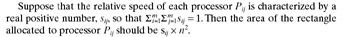The homogeneous two-dimensional block cyclic distribution partitions the matrix into generalized blocks of size (r x m) x (r x m), each partitioned into m x m blocks of the same size r x r, going to separate processors (see Figure 9.2a). The modified, heterogeneous, distribution also partitions the matrix into generalized blocks of the same size, (r x l) x (rx l), where m l n/r. The generalized blocks are identically partitioned into m2 rectangles, each assigned to a different processor. The main difference is that the generalized blocks are partitioned into unequal rectangles. The area of each rectangle is proportional to the speed of the processor that stores the rectangle.

The partitioning of a generalized block can be summarized as follows: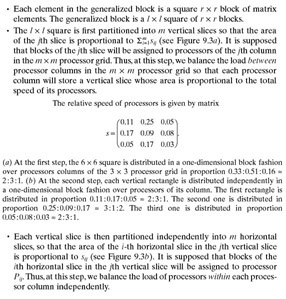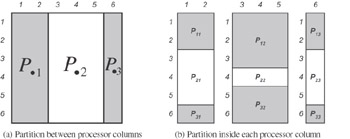Figure 9.3: Example of two-step distribution of a 6 x 6 generalized block over a 3 x 3 processor grid. See below for complete explanation.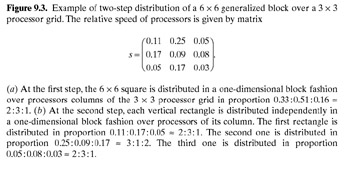Figure 9.4a illustrates the heterogeneous two-dimensional block cyclic distribution from the matrix point of view. Figure 9.4b shows this distribution from the processor point of view. Each rectangle represents the total area of blocks allocated to a single processor.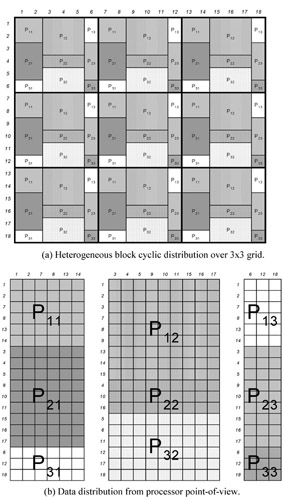Figure 9.4: A matrix with 18 x 18 blocks is distributed over a 3 x 3 processor grid. See below for complete explanation.Figure 9.5 depicts one step of the algorithm of parallel matrix-matrix multiplication on a heterogeneous m x m processor grid. Note that the total volume of communications during execution of this algorithm is exactly the same as that for a homogeneous m x m processor grid. Indeed, at each step k of both algorithms,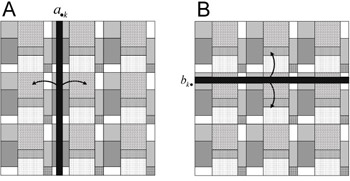Figure 9.5: One step of the algorithm of parallel matrix-matrix multiplication based on heterogeneous two-dimensional block distribution of matrices A, B, and C. First each r x r block of the pivot column a•k of matrix A (shown shaded in dark gray) is broadcast horizontally, and each r x r block of the pivot row bk• of matrix B (shown shaded in dark gray) is broadcast vertically. Then each r x r block cij of matrix C is updated, cij = cij + aik x bkj.

• each r x r block aik of the pivot column of matrix A is sent horizontally from the processor, which stores this block, to m - 1 processors, and

• each r x r block bkj of the pivot row of matrix B is sent vertically from the processor, which stores this block, to m - 1 processors.

The size l of a generalized block is an additional parameter of the heterogeneous algorithm. The range of the parameter is [m, n/r]. The parameter controls two conflicting aspects of the algorithm:

• The level of potential parallelism in execution of successive steps of the algorithm.

The greater is this parameter, the greater is the total number of r x r blocks in a generalized block, and hence the more accurately this number can be partitioned in a proportion given by positive real numbers. Therefore, the greater is this parameter, the better the load of processors is balanced. However, the greater is this parameter, the stronger is the dependence between successive steps of the parallel algorithm, which hinders parallel execution of the steps.

Consider two extreme cases. If l = n/r, the distribution provides the best possible balance of the processors’ load. However, the distribution turns into a pure two-dimensional block distribution resulting in the lowest possible level of parallel execution of successive steps of the algorithm. If l = m, then the distribution is identical to the homogeneous distribution, which does not bother with load-balancing. However, it provides the highest possible level of parallel execution of successive steps of the algorithm. Thus the optimal value of this parameter lies in between these two, being a result of a trade-off between load-balancing and parallel execution of successive steps of the algorithm.

The algorithms are easily generalized for an arbitrary two-dimensional processor arrangement.

9.1.1.3. Implementation of the Heterogeneous Algorithm in mpC. Consider an mpC implementation of the heterogeneous algorithm of parallel matrix multiplication presented in Section 9.1.1.2. One process per physical processor is the assumed configuration of this mpC program. The core of the mpC application is the following definition, which describes the performance model of the algorithm:

` typedef struct {int I; int J;} Processor; nettype ParallelAxB(int m, int r, int N, int l, int w[m],             int h[m][m][m][m]) {    coord I=m, J=m;    node    {       I>=0 && J>=0: bench*(w[J]*h[I][J][I][J]*(N/l)*(N/l)*N);    };    link (K=m, L=m)    {       I>=0 && J>=0 && J!=L && h[I][J][K][L]>0 :          length*(w[J]*h[I][J][K][L]*(N/l)*(N/l)*(r*r)* sizeof(double))             [I, J]->[K, L];       I>=0 && J>=0 && I!=K :          length*(w[I]*h[I][J][I][J]*(N/l)*(N/l)*(r*r)* sizeof(double))             [I, J] -> [K, J];    };    parent[0,0];    scheme    {    int k;    Block Ablock, Bblock;    Processor Root, Receiver, Current;    for(k = 0; k < N; k++)    {       int Acolumn = k%l, Arow;       int Brow = k%l, Bcolumn;       // Broadcast a B row vertically       par(Bcolumn = 0; Bcolumn < l; )       {          GetProcessor(Brow, Bcolumn, &Root);          par(Receiver.I = 0; Receiver.I < m; Receiver.I++)             if(Root.I != Receiver.I)             (100/(h[Root.I][Root.J][Root.I][Root.J]*(N/l)) %%                [Root.I, Root.J]->[Receiver.I, Root.J];          Bcolumn += w[Root.J];    }    // Brodacast an A column horizontally    par(Arow = 0; Arow < l; )    {       GetProcessor(Arow, Acolumn, &Root);       par(Receiver.I = 0; Receiver.I < m; Receiver.I++)          par(Receiver.J = 0; Receiver.J < m; Receiver.J++)             if((Root.I != Receiver.I || Root.J !=                 Receiver.J) && Root.J != Receiver.J)             if(h[Root.I][Root.J][Receiver.I][Receiver.J])             (100/(w[Root.J]*(N/l)))%%                [Root.I, Root.J]->[Receiver.I, Receiver.J];    Arow += h[Root.I][Root.J][Root.I][ Root.J];          }          // Update C matrix          par(Current.I = 0; Current.I < m; Current.I++)             par(Current.J = 0; Current.J < m; Current.J++)             (100/N) %% [Current.I, Current.J];       }    }; };`

The network type ParallelAxB describing the algorithm has six parameters. Parameter m specifies the number of abstract processors along the row and along the column of the processor grid executing the algorithm. Parameter r specifies the size of a square block of matrix elements, the updating of which is the unit of computation of the algorithm. Parameter N is the size of square matrices A, B, and C measured in r x r blocks. Parameter l is the size of a generalized block also measured in r x r block.

Vector parameter w specifies the widths of the rectangles of a generalized block assigned to different abstract processors of the m x m grid. The width of the rectangle assigned to processor PIJ is given by element w[J] of the parameter (see Figures 9.3b and 9.4b). All widths are measured in r x r blocks.

Parameter h specifies the heights of rectangle areas of a generalized block of matrix A, which are horizontally communicated between different pairs of abstract processors. Let RIJ and RKL be the rectangles of a generalized block of matrix A assigned to processors PIJ and PKL respectively. Then h[I][J][K][L] gives the height of the rectangle area of RIJ, which is required by processor PKL to perform its computations. All heights are measured in r x r blocks.

Figure 9.6 illustrates possible combinations of rectangles RIJ and RKL in a generalized block. Let us call a r x r block of RIJ a horizontal neighbor of RKL if the row of r x r blocks that contains this r x r block will also contain a r x r block of RKL. Then the rectangle area of RIJ, which is required by processor PKL to perform its computations, comprises all horizontal neighbors of RKL.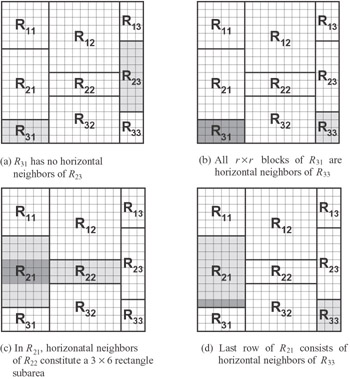Figure 9.6: Different combinations of rectangles in a generalized block. (a) No r x r block of rectangle R31 is a horizontal neighbor of rectangle R23; therefore h = 0. (b) All r x r blocks of rectangle R31 are horizontal neighbors of rectangle R33; h = 3. (c) Neighbors of rectangle R22 in rectangle R21 make up a 3 x 6 rectangle area (shaded in dark gray); h = 3. (d) Neighbors of rectangle R33 in rectangle R21 make up the last row of this rectangle (shaded in dark gray); h = 1.

Figure 9.6a shows the situation where rectangles RIJ and RKL have no horizontal neighbors. Correspondingly h[I][J][K][L] is zero. Figure 9.6b shows the situation where all r x r blocks of RIJ are horizontal neighbors of RKL. In this case both h[I][J][K][L] are equal to the height of RIJ. Figures 9.6c and 9.6d show the situation where only some of r x r blocks of RIJ are horizontal neighbors of RKL. In this case h[I][J][K][L] is equal to the height of the rectangle subarea of RIJ comprising the horizontal neighbors of RKL. Note that h[I][J][I][J] specifies the height of RIJ, and h[I][J][K][L] will be always equal to h[K][L][I][J].

The coord declaration introduces two coordinate variables, I and J, both ranging from 0 to m - 1. The node declaration associates abstract processors with this coordinate system to form a m x m grid. It also describes the absolute volume of computation to be performed by each of the processors. As a unit of measure, the volume of computation performed by the code multiplying two r x r matrices is used. At each step of the algorithm, abstract processor PIJ updates (wIJ x hIJ) x ng r x r blocks, where wIJ, hIJ are the width and height of the rectangle of a generalized block assigned to processor PIJ , and ng is the total number of generalized blocks. As computations during the updating of one r x r block mainly fall into the multiplication of two r x r blocks, the volume of computations performed by the processor PIJ at each step of the algorithm will be approximately (wIJ x hIJ) x ng times larger than the volume of computations performed to multiply two r x r matrices. As wIJ is given by w[J], hIJ is given by h[I][J][I][J], ng is given by (N/l)*(N/l), and the total number of steps of the algorithm is given by N, the total volume of computation performed by abstract processor PIJ will be w[J]*h[I][J][I][J]*(N/l)*(N/l)*N times bigger than the volume of computation performed by the code multiplying two r x r matrices.

The link declaration specifies the volumes of data to be transferred between the abstract processors during the execution of the algorithm. The first statement in this declaration describes communications related to matrix A. Obviously abstract processors from the same column of the processor grid do not send each other elements of matrix A. Abstract processor PIJ will send elements of matrix A to processor PKL only if its rectangle RIJ in a generalized block has horizontal neighbors of the rectangle RKL assigned to processor PKL. In this case processor PIJ will send all such neighbors to processor PKL. Thus, in total, processor PIJ will send NIJKL x ng r x r blocks of matrix A to processor PKL, where NIJKL is the number of horizontal neighbors of rectangle RKL in rectangle RIJ and ng is the total number generalised blocks. As NIJKL is given by w[J]* h[I][J][K][L], ng is given by (N/l)*(N/l), and the volume of data in one r x r block is given by (r*r)*sizeof(double), the total volume of data transferred from processor PIJ to processor PKL will be given by w[J]*h[I][J][K][L]*(N/l)*(N/l)*(r*r)*sizeof(double).

The second statement in the link declaration describes communications related to matrix B. Obviously only abstract processors from the same column of the processor grid send each other elements of matrix B. In particular, processor PIJ will send all of its r x r blocks of matrix B to all other processors from column J of the processor grid. The total number of r x r blocks of matrix B assigned to processor PIJ is given by w[J]*h[I][J][I][J]*(N/l)*(N/l).

The scheme declaration describes n successive steps of the algorithm. At each step k:

• A row of r x r blocks of matrix B is communicated vertically. For each pair of abstract processors PIJ and PKJ involved in this communication, PIJ sends a part of this row to PKJ. The number of r x r blocks transferred from PIJ to PKJ will be , where is the number of generalized blocks along the row of r x r blocks. The total number of r x r blocks of matrix B, which processor PIJ sends to processor PKJ, is (wIJ x hIJ) x ng. Thereforepercent of data that should be in total sent from processor PIJ to processor PKJ will be sent at this step. The first nested par statement in the main for loop of the scheme declaration just specifies it. The par algorithmic patterns are used to specify that during the execution of this communication, data transfer between different pairs of processors is carried out in parallel.

• A column of r x r blocks of matrix A is communicated horizontally. If processors PIJ and PKL are involved in this communication so that PIJ sends a part of this column to PKL, then the number of r x r blocks transferred from PIJ to PKL will be , where HIJKL is the height of the rectangle area in a generalized block, which is communicated from PIJ to PKL, and is the number of generalized blocks along the column of r x r blocks. The total number of r x r blocks of matrix A that processor PIJ sends to processor PKL, is NIJKL x ng. Thereforepercent of data that should be in total sent from processor PIJ to processor PKL will be sent at this step. The second nested par statement in the main for loop of the scheme declaration specifies this fact. Again, we use the par algorithmic patterns in this specification to stress that during the execution of this communication, data transfer between different pairs of processors is carried out in parallel.

• Each abstract processor updates each its r x r block of matrix C with one block from the pivot column and one block from the pivot row so that each block cij (i, j {1, . . ., N}) of matrix C is updated, cij = cij + aik x bkj. The processor performs the same volume of computation at each step of the algorithm. Therefore at each of N steps of the algorithm the processor will perform 100/N% of the volume of computations it performs during the execution of the algorithm. The third nested par statement in the main for loop of the scheme declaration just says so. The par algorithmic patterns are used here to specify that all abstract processors perform their computations in parallel.

Function GetProcessor is used in the scheme declaration to iterate over abstract processors that store the pivot row and the pivot column of r x r blocks. It returns in its third parameter the grid coordinates of the abstract processor storing the r x r block, whose coordinates in a generalized block of a matrix are specified by its first two parameters.

The main function of this application appears as follows:

`#define H(a, b, c, d, m) h[(a*m*m*m+b*m*m+c*m+d)] int [*] main(int [host] argc, char** [host] argv)    {       repl int m, r, N, l, *w, *h, *trow, *lcol;       repl double* speeds;       int [host] opt_l;       double [host] time_i, Elapsed_time;       m = [host]atoi(argv);       r = [host]atoi(argv);       N = [host]atoi(argv);       // Update the estimation of the processors speed       {          repl int i, j;          repl double x[r*r], y[r*r], z[r*r];          for(i = 0; i < r; i++)             for(j = 0; j < r; j++) {                x[i*r+j] = 2.;                y[i*r+j] = 2.;                z[i*r+j] = 0.;             }          recon rMxM(x, y, z, r);       }          // Detect the speed of the physical processors          speeds = (double*)malloc(sizeof(double)*m*m);          MPC_Get_processors_info(NULL, speeds);          // w --- widths of rectangular areas in generalized block          // h --- heights of ‘horizontal-neighbors’ subrectangles          // trow --- Top rows of rectangular areas in generalized block          // lcol --- Left columns of these rectangular areas          w = (int*)malloc(sizeof(int)*m);          h = (int*)malloc(sizeof(int)*(m*m*m*m));          trow = (int*)malloc(sizeof(int)*m*m);          lcol = (int*)malloc(sizeof(int)*m);          // Determine the optimal generalized block size          [host]:          {             int i;             double algo_time, min_algo_time = DBL_MAX;             for(i = m; i < N; i++) {                DistributeLoad(m, speeds, i, w, h, trow, lcol);                algo_time = timeof(net ParallelAxB(m, r, N, i, w, h) paxb);                if(algo_time < min_algo_time) {                   opt_l = i;                   min_algo_time = algo_time;                }             }          }          // Start timing the algorithm          time_i = [host]MPC_Wtime();          // Broadcast the optimal generalized block size          l = opt_l;          // Determine widths, top rows, top columns of the rectangular          // areas in generalized block, and the heights of their          // ‘horizontal-neighbors’ subrectangles          DistributeLoad(m, speeds, l, w, h, trow, lcol);          free(speeds);          {             // Create the mpC network             net ParallelAxB(m, r, N, l, w, h) g;             int [g] icrd, [g] jcrd;             // Initialize the matrix elements and execute the algorithm             [g]:             {             int x, y, i, j, s, t, MyTopRow, MyLeftColumn;             double *a, *b, *c;             a = (double*)malloc(sizeof(double)*(N*r)*(N*r));             b = (double*)malloc(sizeof(double)*(N*r)*(N*r));             c = (double*)malloc(sizeof(double)*(N*r)*(N*r));             icrd = I coordof g;             jcrd = J coordof g;             MyTopRow = trow[icrd*m + jcrd];             MyLeftColumn = lcol[jcrd];             for(x = MyTopRow; x < N; x+=l)                for(y = MyLeftColumn; y < N; y+=l)                      for(i = 0; i < H(icrd,jcrd,icrd,jcrd,m); i++)                   for(j = 0; j < w[jcrd]; j++)                   for(s = 0; s < r; s++)                   for(t = 0; t < r; t++) {                          a[(x*r*N*r)+y*r+(i*r*N*r)+j*r+(s*r*N)+t] = 2.;                          b[(x*r*N*r)+y*r+(i*r*N*r)+j*r+(s*r*N)+t] = 2.;                          c[(x*r*N*r)+y*r+(i*r*N*r)+j*r+(s*r*N)+t] = 0.;                      }                ([(m,r,N,l,w,h)g])ComputeAxB(icrd,jcrd,a,b,c,trow,lcol);                free(w); free(h); free(trow); free(lcol);                free(a); free(b); free(c);                ([(0)g])MPC_Barrier();             }          }          // Print the algorithm execution time          [host]:          {             Elapsed_time = MPC_Wtime() - time_i;             printf("Problem size=%d, time(sec)=%0.6f, time(min)=%0.6f\n",                               N*r, Elapsed_time,                       Elapsed_time/60.);    } }`

This code specifies the following sequence of steps performed by the mpC program:

• First, it gets the inputs to the mpC application. These inputs are

• the size of block stored in the replicated variable r,

• the problem size stored in the replicated variable N, and measured in r x r blocks, and

• the number of processors along a row or column stored in the replicated variable m.

• Then, the actual speeds of the processors are estimated with the help of the recon statement. This recon statement uses a call to function rMxM, which multiplies two r x r matrices, as a test code.

• Then, function MPC_Get_processors_info is used to obtain the actual processors speeds and store them in the replicated array speeds.

• Then, the optimal generalized block size is computed on the host-process with the help of the timeof operator.

• Then, the optimal generalized block size is broadcast from the host-process to all the other processes of the parallel program.

• Then, the parameters of the heterogeneous parallel algorithm are computed in parallel by all processes of the mpC program, using function DistributeLoad. This function calculates the parameters of the heterogeneous block distribution of a generalized l x l block across an m x m grid of processors in proportion to their speeds given by elements of array speeds.

• Then, mpC network g of type ParallelAxB is created using the computed parameters of the heterogeneous algorithm. Abstract processors of this mpC network are mapped to the processes of the program so as to minimize the algorithm execution time. As one process per physical processor is supposed, there will be one-to-one mapping between the abstract processors of the mpC network g and the physical processors of the executing network.

• Then, the matrix elements are initialized on the abstract processors of network g.

• Then, all the abstract processors of network g call the network function ComputeAxB to perform the core computations and communications of the algorithm.

• Then, the elapsed execution time is printed on the console associated with the host-processor.

The full text of the mpC program is given in Appendix B. In particular, it includes the source code for functions DistributeLoad and GetProcessor. Actually mpC provides the library functions for an optimal distribution of datasets and workloads in heterogeneous environments for a wide range of applications, and we could use calls to some of the functions instead of calls to DistributeLoad and GetProcessor. The reason behind our using home-made functions is to give an idea of how the mpC distribution library functions are implemented.

9.1.1.4. Experimental Results. We will assess the heterogeneous algorithm by comparing with its homogeneous prototype. Thus we will assess the heterogeneous modification rather than analyze the algorithm as an isolated entity. Our basic postulate is that the heterogeneous algorithm cannot be more efficient than its homogeneous prototype. By this we mean that the heterogeneous algorithm cannot be executed on the heterogeneous network faster than its homogeneous prototype on the equivalent homogeneous network. A homogeneous network of computers is equivalent to the heterogeneous network if

• its communication characteristics are the same,

• it has the same number of processors, and

• the speed of each processor is equal to the average speed of processors of the heterogeneous network.

The heterogeneous algorithm is considered optimal if its efficiency is the same as that of its homogeneous prototype. To compare the heterogeneous algorithm of matrix multiplication with its homogeneous prototype, we assume that parameters n, m, and r are the same. Then both algorithms consist of n/r successive steps.

At each step, equivalent communication operations are performed by each of the algorithms:

• Each r x r block of the pivot column of matrix A is sent horizontally from the processor that stores this block to m - 1 processors.

• Each r x r block of the pivot row of matrix B is sent vertically from the processor stores this block to m - 1 processors.

Thus the per-step communication cost is the same for both algorithms.

If l is large enough, then at each step each processor of the heterogeneous network will perform the volume of computation approximately proportional to its speed. In this case the per-processor computation cost will be approximately the same for both algorithms.

Thus the per-step cost of the heterogeneous algorithm will be approximately the same as that of the homogeneous one. So the only reason for the heterogeneous algorithm to be less efficient than its homogeneous prototype is a lower level of potential overlapping of communication operations at successive steps of the algorithm. Obviously the bigger the ratio between the maximal and minimal processor speed, the lower is this level. Note that if the communication layer serializes data packages (e.g., plain Ethernet), then the heterogeneous algorithm has approximately the same efficiency as the homogeneous one. Therefore in this case the presented heterogeneous algorithm is the optimal modification of its homogeneous prototype.

In this section we present some experimental results that allow us to estimate the significance of the additional dependence between the successive steps of the algorithm if the communication layer allows multiple data packages. Now we look at some results of experiments with this mpC application. All the results are obtained for r=8 and generalized block size l=9, which seem to be optimal for both homogeneous and heterogeneous block cyclic distributions.

A small heterogeneous local network of nine different Solaris and Linux workstations is used in the experiments presented in Figure 9.7. The relative speed of the workstations demonstrated on the core computation of the algorithms (updating of a matrix) is as follows: 46, 46, 46, 46, 46, 46, 46, 84, and 9. The network is based on 100 Mbit Ethernet with a switch enabling parallel communications among the computers.Figure 9.7: Execution times of the heterogeneous and homogeneous 2D algorithms and the heterogeneous 1D algorithm. All algorithms are performed on the same heterogeneous network.

For the experiments presented in Figure 9.8 we use the same heterogeneous network and a homogeneous local network of nine Solaris workstations with the following relative speeds: 46, 46, 46, 46, 46, 46, 46, 46, 46. The two sets of workstations share the same network equipment. Note that the total performance of the processors of the heterogeneous network is practically the same as that of the homogeneous one.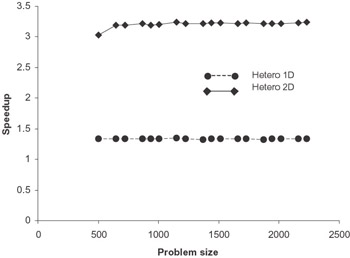Figure 9.8: Speedups of the heterogeneous 1D and 2D algorithms compared with that of the homogeneous 2D block cyclic algorithm. All algorithms are performed on the same heterogeneous network.

Figure 9.7 shows the comparison of the execution times of three parallel algorithms of matrix multiplication:

• The algorithm based on 2D heterogeneous block cyclic distribution.

• The algorithm based on 1D heterogeneous block cyclic distribution.

• The algorithm based on 2D homogeneous block cyclic distribution.

Clearly, the 2D heterogeneous algorithm is almost twice as fast as the 1D heterogeneous algorithm and almost three times as fast as the 2D homogeneous one. Figure 9.8 compares the speedups by the heterogeneous and the homogeneous algorithms. Figure 9.9 compares the execution times of the 2D heterogeneous block cyclic algorithm performed on the heterogeneous network and the 2D homogeneous block cyclic algorithm performed on the homogeneous network.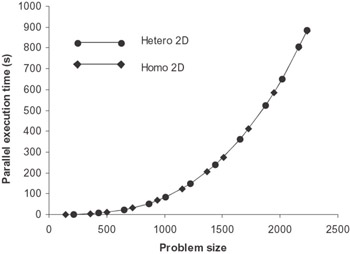Figure 9.9: Execution times of the 2D heterogeneous block cyclic algorithm on a heterogeneous network and of the 2D homogeneous block cyclic algorithm on a homogeneous network. The networks have approximately the same total power of processors and share the same communication network.

We can see that the algorithms demonstrate practically the same speed but each on its own network. As the two networks are practically of the same power, we can conclude that the heterogeneous algorithm is very close to the optimal heterogeneous modification of the basic homogeneous algorithm. The experiment shows that the additional dependence between successive steps introduced by the heterogeneous modification has practically no impact on the efficiency of the algorithm. This may be explained by the following two facts:

• The speedup due to the overlapping of communication operations performed at successive steps of the algorithm is not particularly significant.

• The speeds of processors in the heterogeneous network do not differ much. Actually the network is moderately heterogeneous. Therefore, for this particular network, the additional dependence between steps is very weak.

Thus, for reasonably heterogeneous networks, the presented heterogeneous algorithm has proved to be very close to the optimal one by significantly accelerating matrix multiplication on such platforms compared to its homogeneous prototype.

### 9.1.2. Matrix Factorization

Many complex scientific and engineering problems involve solutions of large linear systems of equations as the key computational steps. LU, QR, and Cholesky factorizations are the most widely used methods for solving dense linear systems of equations, and they have been extensively studied and implemented on serial and parallel computers.

In this section we first introduce Cholesky factorization and a parallel algorithm for Cholesky factorization on homogeneous distributed memory multiprocessors. Then we give a modification of this algorithm for heterogeneous networks of computers, outline its implementation in the mpC language, and present some experimental results.

9.1.2.1. The Cholesky Factorization. We start introducing the Cholesky factorisation from some basic definitions. A real nx n matrix A is symmetric if A = AT. A quadratic form is a function f:n that can be expressed as where A = AT. A is positive definite if xT Ax > 0 for all x 0.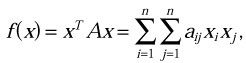Now we can formulate main properties of positive definite matrices. Let A be a real, n x n, symmetric, positive definite matrix.

Property 1. A is nonsingular, namely Ax 0 for all nonzero x.

 Proof. By definition, xT Ax > 0 for all nonzero x; hence Ax≠ 0. ￭

Property 2. aii > 0 for all i.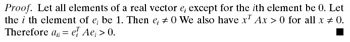Property 3. If A is partitioned as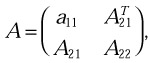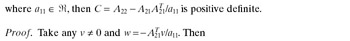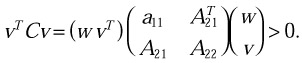Thus, if A is a real, symmetric, positive definite matrix, then it can be factorized as A = LLT, where L is lower triangular with positive diagonal elements. This factorization is called the Cholesky factorization of A. ￭

The recursive algorithm for implementing the factorization is derived from the obvious equations: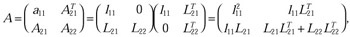where a11 and l11 are scalars, A21 and L21 are vectors of length n - 1, and A22 and L22 are matrices of size (n - 1) x (n - 1). From this we derive the equations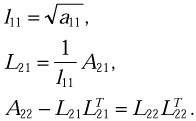The algorithm for computing the Cholesky factorization can be summarized as follows: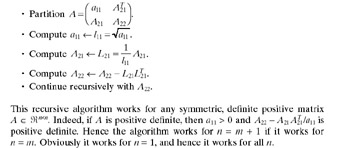Solving a positive-definite linear system of equations

Ax =b

where A = AT nn and positive definite, can be carried out as follows:

• First, A is factorized as A = LLT.

• Then, LLTx = b is solved in two steps:

• First, by forward substitution Lz = b.

• Second, by backward substitution LTx = z.

9.1.2.2. Block Cyclic Algorithm of Parallel Cholesky Factorization on Homogeneous Platforms. The serial algorithm of Cholesky factorization presented in Section 9.1.2.1 factors a n x n matrix A into the product of a lower triangular matrix L and its transpose in n successive steps. At each step the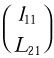column is computed and the trailing submatrix A22 is updated. It is assumed that the computed elements of L overwrite the given elements of A.

A block-partitioned form of this algorithm allows for more effective use of a memory hierarchy. Each element in A is now a square r x r block. For simplicity we assume that n is a multiple of r. The block Cholesky factorization algorithm takes n/r steps and is derived from the following equations: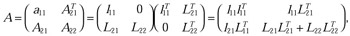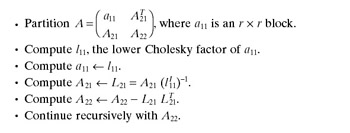where a11 and l11 are r x r blocks, A21 and L21 are of size (n - r) x r, and A22 and L22 are matrices of size (n - r) x (n - r). L22 and l11 are lower triangular. If we assume that l11, the lower triangular Cholesky factor of a11, is known, we can compute L21,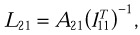and update A22,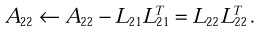Thus the block Cholesky factorization algorithm can be summarized as follows:Figure 9.10 shows one step of this algorithm. At each step the column panel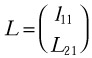is computed, and the trailing submatrix A22 is updated.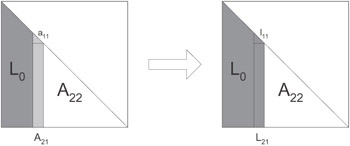Figure 9.10: One step of the block Cholesky factorization algorithm: See below for complete explanation.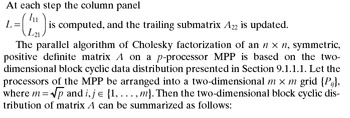• Each element in A is a square r x r block.

• The blocks are scattered in a cyclic fashion along both dimensions of the m x m processor grid, so that for each block aij will be mapped to processor PIJ so that I = (i - 1) mod m + 1 and J = (j - 1) mod m + 1 (see Figure 9.2).

One step of the parallel Cholesky factorization algorithm proceeds as follows:

• First a processor that has the r x r diagonal block a11 performs the Cholesky factorization of a11 computing its lower triangular factor l11.

• Then l11 is broadcast vertically along a column of blocks A21 on the processors that have this column.

• The processors of this grid column compute a column of blocks L21.

• The column of blocks L21 is broadcast horizontally so that each r x r block of this column is broadcast along a row of the processor grid. Now each processor has its own portion of L21.

• For processors to have portions of LT21, the row of blocks LT21 should be first computed and then broadcast vertically so that each r x r block of this row is broadcast along a column of the processor grid. This operation is performed more efficiently if each r x r block L21 is broadcast to all processors of the corresponding grid column, and then locally transposed. Now each processors has its own portions of L21 and LT21.

• Finally all processors update their local portions of the matrix A22.

Cholesky factorization demonstrates the advantage of the block cyclic data distribution over the block data distribution more explicitly than matrix multiplication. Indeed, at each next step of the Cholesky factorization, a matrix of smaller size is processed (see Figure 9.10). At the first step, we process a matrix of size n x n. At the second step, it is a matrix of size (n - r) x (n - r). At the step k, a matrix of size (n - (k - 1) x r) x (n - (k - 1) x r) is processed. Therefore, if matrix A is block distributed, then the number of processors involved in the execution of the algorithm will decrease with the algorithm’s progression. Namely the first n/(m x r) steps of the two-dimensional block algorithm will be executed by all processors of the m x m grid. The next n/(m x r) steps will not involve the first row and first column of the processor grid. The next n/(m x r) steps will not involve the processors of the first two rows and first two columns of this grid, and so on. This results in an unbalanced load of processors during the algorithm’s execution. In contrast, the two-dimensional block cyclic distribution allows all processors of the grid to evenly load through most of the algorithm’s execution.

9.1.2.3. Block Cyclic Cholesky Factorization on Heterogeneous Platforms. The algorithm of Cholesky factorization on a heterogeneous cluster is obtained from the parallel Cholesky factorization algorithm presented in Section 9.1.2.2 by using a heterogeneous block cyclic distribution of matrix A (see Section 9.1.1.2) instead of a homogeneous one. Both homogeneous and heterogeneous block cyclic algorithms are easily generalized for an arbitrary two-dimensional processor arrangement.

The heterogeneous parallel algorithm of Cholesky factorization was implemented in mpC. The key fragments of the parallel mpC code are as follows:

` nettype HeteroGrid (int P, int Q, double grid[P*Q]) {    coord I=P, J=Q;    node {I>=0 && J>=0: grid[I*Q+J];}; }; ... repl P, Q, PxQ; repl double *speeds; ... {    int n=100, info;    double a[n][n];    Initialize(a, n);    recon dpotf2_("U", &n, a, &n, &info); } MPC_Get_processors_info(&PxQ, speeds); MakeGrid(PxQ, &P, &Q); {    net HeteroGrid (P, Q, speeds) phf;    ... }`

In this mpC code the network type definition supplies a simple performance model of the implemented parallel algorithm. It introduces

• The name HeteroGrid of the network type.

• A list of parameters, including integer scalar parameters P, Q, and vector parameter grid of P*Q floating-point elements.

• Coordinate variables, I, ranging from 0 to P-1, and J, ranging from 0 to Q-1.

• The abstract processors performing the algorithm are associated with this coordinate system, and the relative volume of computations to be performed by each processor is declared. The abstract processors are arranged into a P x Q grid. The relative area of r x r blocks mapped to the (I, J)-th processor of this grid is given by grid[I*Q+J].

It is assumed that the program is executed by a heterogeneous cluster with one process per processor running, so that there is always one-to-one mapping between abstract and physical processors. The recon statement uses the LAPACK routine dpotf2 performing serial Cholesky factorization of a 100 x 100 dense matrix to update the speed estimation of each physical processor.

The library function MPC_Get_processors_info returns the number of available physical processors (in the variable PxQ) and their relative speeds (in the array speeds). Function MakeGrid suggests a two-dimensional arrangement of the processors returning the number of processor rows in variable P, and the number of processor columns in variable Q.

The next key line of this code defines the abstract network phf of the type HeteroGrid, with the actual parameters P and Q specifying the actual size of the physical processor grid, and speeds, an array of the relative speeds of the physical processors. The remaining computations and communications will be performed on this abstract network.

The mpC programming system maps abstract processors of network phf to physical processors so that the total area of blocks of matrix A mapped to each physical processor will be proportional to its relative speed. Therefore the load of processors will be balanced during the program execution.

Now we turn to the results of some experiments with Cholesky factorization on a local network of eight Sun workstations interconnected via plain 10 Mbit Ethernet. Table 9.1 gives the relative speeds demonstrated by the workstations upon execution of a serial Cholesky factorization of a 100 x 100 dense matrix with the LAPACK routine dpotf2.

Table 9.1: Relative speeds of workstations demonstrated on serial Cholesky factorization

Workstation’s Number

Relative Speed

1–4

1.9

5

1.0

6–7

2.8

8

7.1

In the experiments the homogeneous and heterogeneous block cyclic parallel algorithms of the Cholesky factorization were compared. The workstations were logically arranged into a 2 x 4 grid. The homogeneous algorithm was executed with block size r = 6, and the heterogeneous algorithm with block size r = 20. Block sizes were found that experimentally provided the best execution time for each algorithm.

Figure 9.11 shows that the heterogeneous algorithm speeds up the Cholesky factorization on the heterogeneous network of workstations compared to its homogeneous counterpart. This speedup is mainly due to better load balancing. Note that the speedup is relatively low compared with the experimental results for matrix multiplication in Section 9.1.14. It is explained by the larger contribution of communication operations into the total execution time: due to the nature of Cholesky factorization, on the one hand, and the low performance of the network, on the other.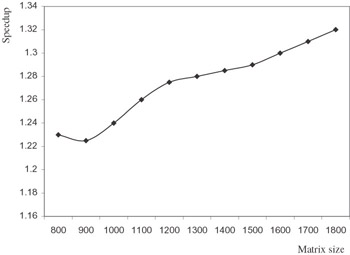Figure 9.11: Speedup achieved by the heterogeneous block cyclic Cholesky factorization compared to its homogeneous counterpart on a heterogeneous network of eight workstations.

### 9.1.3. Heterogeneous Distribution of Data and Heterogeneous Distribution of Processes Compared

In Sections 9.1.1 and 9.1.2 we designed parallel algorithms that solve regular problems such as dense linear algebra problems on heterogeneous networks. This approach can be summarized as follows:

• Start with a parallel algorithm that solves the problem on MPPs. This algorithm should evenly distribute data and, hence, computations across parallel processes, with one process per processor running.

• Then modify this homogeneous algorithm by unevenly distributing data across processes so that each process performs the volume of computations proportional to the speed of the processor running this process. One process is to run per processor.

In this section we present another approach in our design of parallel algorithms for regular problems on heterogeneous networks. We again start with a homogeneous parallel algorithm that solves the problem on MPPs. The modified algorithm distributes data across parallel processes exactly in the same fashion as its homogeneous prototype. However, this algorithm is modified to allow: more than one process involved in its execution to be run on each processor so that the number of processes running on the processor is proportional to its speed. In other words, while distributed evenly across parallel processes, data and computations are distributed unevenly over processors of the heterogeneous network, and this way each processor performs the volume of computations proportional to its speed.

The mpC language provides natural implementation in a portable form of heterogeneous algorithms of this type. Consider how the corresponding algorithm of the Cholesky factorization can be implemented in mpC:

` nettype Grid(int P, int Q) {    coord I=P, J=Q; }; int [net Grid(int p, int q) w]mpC2Cblacs_gridinit(int*, char*); void [*]Cholesky(repl int P, repl int Q) {    {       int n=100, info;       double a[n][n];       Initialize(a, n);       recon dpotf2_("U", &n, a, &n, &info);    }    {       net Grid (P, Q) phf;       [phf]:       {          int ConTxt;          ([(P,Q)phf])mpC2Cblacs_gridinit(&ConTxt, "R");          pdlltdriver1_(&ConTxt);          mpC2Cblacs_gridexit(ConTxt);       }    } }`

In this code, the definition of the network type Grid describes the simplest performance model of the implemented parallel algorithm when all abstract processors perform the same volume of computations.

It is assumed that the program is executed by a heterogeneous cluster, with each processor running several processes. The recon statement uses the LAPACK routine dpotf2 performing serial Cholesky factorization of a 100 x 100 dense matrix to update the speed estimation of each physical processor.

The mpC network phf executing the parallel algorithm is then defined as consisting of P*Q abstract processors each performing the same volume of computations. The abstract processors will be mapped to the parallel processes of the program so that the number of abstract processors mapped to each physical processor is proportional to the processor’s speed.

The parallel Cholesky factorization is actually performed on this mpC network by calls to ScaLAPACK routines. ScaLAPACK is a well-known library for solving linear algebra problems on MPPs. The BLACS (Basic Linear Algebra Communication Subprograms) is a communication layer of ScaLAPACK providing a linear algebra oriented message-passing interface.

In the BLACS there are two routines, Cblacs_gridinit and Cblacs_gridmap, that create a process grid and its enclosing context. The context is an analogue of the MPI’s communicator. These routines return context handles that are simple integers. Subsequent BLACS routines will be passed these handles, which allow the BLACS to determine what grid a routine is being called from. The routine Cblacs_gridexit releases contexts. The mpC network function

` int_[net_Grid(int_p,_int_q)_w]mpC2Cblacs_gridinit(int_*pConTxt,    char *order)`

is a wrapper for the BLACS routine

` int Cblacs_gridinit(int * pConTxt, char * order,     int p, int q) ,`

where pConTxt is a pointer to the context to be created, p and q are numbers of rows and columns in the process grid associated with the context, and order indicates how to map processes to the BLACS grid. Thus the mpC function mpC2Cblacs_gridinit creates the BLACS grid and associated context from processes representing abstract processors of the mpC network w. The created context can be used to call ScaLAPACK routines. The mpC function mpC2Cblacs_gridexit is an mpC wrapper for the ScaLAPACK function Cblacs_gridexit.

Thus, in the mpC code above, a call to function mpC2Cblacs_gridinit creates the BLACS context associated with abstract processors of the mpC network phf. In this context the ScaLAPACK test driver for Cholesky factorization is called on the network phf. This driver reads problem parameters (matrix and block sizes) from a file, forms a test matrix, and performs its Cholesky factorization.

The presented approach has the advantage of allowing us to easily utilize parallel software that has been developed for MPPs. The disadvantage is that it requires a larger number of processes, so more resources are consumed and more inter-process communications created. This will increase the overhead, although, in many instances the additional overhead has not been significant. Figure 9.12 compares two such mpC applications performing Cholesky factorization on the same heterogeneous network of eight workstations as are described in Section 9.1.2. The first application (presented in Section 9.1.2) is based on a heterogeneous distribution of data across processes with one process per processor running; the second (presented in this section) is based on heterogeneous distribution of a larger number of evenly loaded processes across the processors. As can be seen the first application is faster, but the difference is not very big and it lessens with growth of matrix size.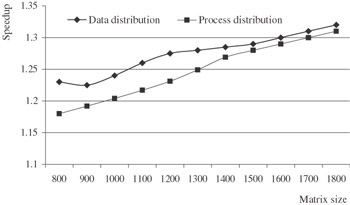Figure 9.12: Speedups achieved by the heterogeneous Cholesky factorizations based on the data distribution and process distribution compared to their homogeneous prototype on a heterogeneous network of eight workstations.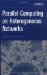Parallel Computing on Heterogeneous Networks (Wiley Series on Parallel and Distributed Computing)
ISBN: B000W7ZQBO
EAN: N/A
Year: 2005
Pages: 95